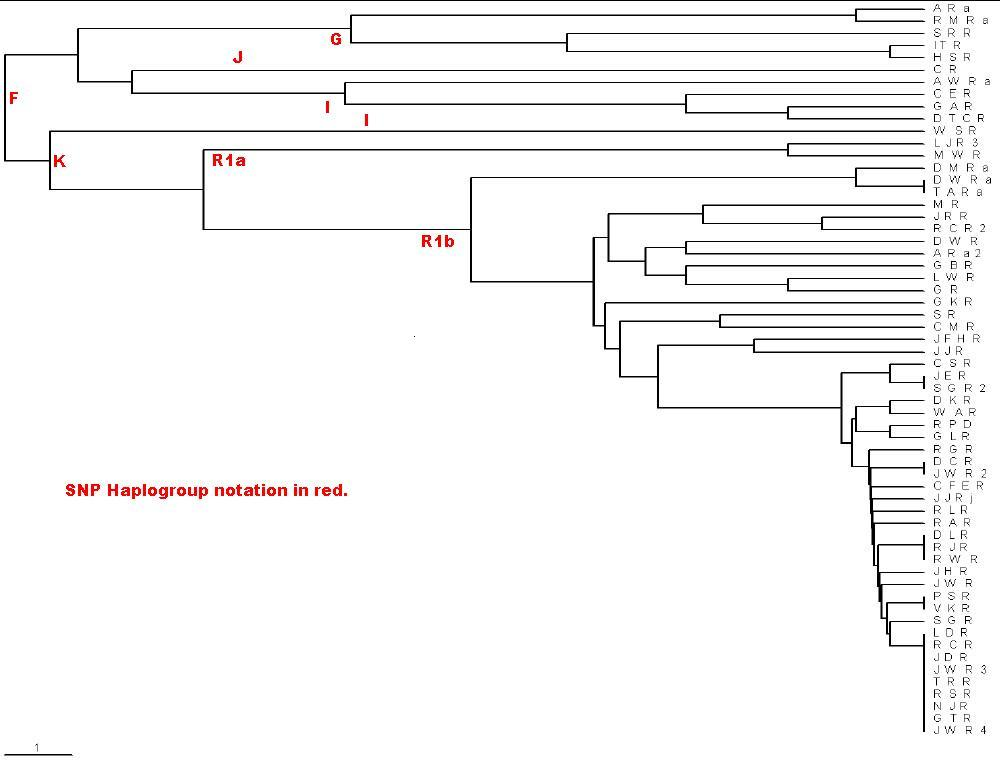### Brief Instructions for Using the PHYLIP/Neighbor or Kitsch Programs to Calculate and the TreeView Program to Plot Phylograms

• PHYLIP software. Only the Neighbor or Kitsch program is used. The Neighbor program can be much faster than the Kitsch program, but it is not as accurate. (I have tested the two programs and found that, indeed, Neighbor is not very accurate even for a small number of persons. The Neighbor program computer time increases as the square of the number of persons and the Kitsch computer time increases as the fourth power of the number of persons. I usually use Neighbor because it is much faster and is accurate enough for my purposes.
• TreeView software

The following rules describe how I use the Neighbor or the Kitsch program of PHYLIP to calculate a phylogram tree file for the 25 Y-chromosome markers:

• Use MS Excel to create a relative-mutations matrix for the set of 25-markers for which I want a phylogram.
• Copy the relative-mutations matrix and then paste it into MS NotePad. (Do not use MS Word, because you will get a table instead of text.) There will be tabs between each element of the matrix.
• Count the number of lines in the relative-mutations matrix and type that number in the first line of the file.
• There must be exactly ten characters or spaces at the beginning of each line after the first line. Replace the tab after the name at the beginning of each line of the matrix with enough spaces to make ten characters or spaces.
• If you name the file infile in the same directory as the Neighbor or Kitsch program is in, the program will use that file when you run it. I prefer to create a directory called Data, in the directory of the Neighbor program, in which to put the data file and to give it a descriptive name.
• Run the Neighbor or Kitsch program. (I put a shortcuts for them on the desktop.) When it asks for a data file, type Data\<yourfilename>.
• Then for the Neighbor program type N to use the UPGMA option (Unweighted Pair Group Method with Arithmetic Mean).
• Then type L to use lower-triangular matrix input or R to use upper-triangular matrix input, depending on how you arrange the relative-mutations matrix.
• It is recommended that the Jumble option be used to increase the probability of finding the best tree. If you select J you will be asked to input a random-number seed and how many times to jumble the input; ten times is recommended.
• Then type Y to do the calculation. After the calculation the Neighbor program will close itself.
• The calculation creates two files in the directory with the Neighbor program: outfile and treefile. Ignore the outfile.
• Create a directory Tree under the Neighbor directory and move treefile to that directory. Rename treefile to a descriptive name with a tre suffix; e.g. Roper.tre .
• If the TreeView program installed correctly, all you need to do now is just click on the *.tre file to put it into the TreeView program.
• After the tree is shown in TreeView, choose the Tree-Phylogram option.
• Now do File-Save as graphic and choose the *.wmf type of graphics file. Use some graphics program to convert this *.wmf file to *.jpg or *.gif .
• Now you have a file that you can use in a document or on a web page.

For another description of the procedure given above see CalculateAndPlotPhylograph.pdf .

Here is an example input file for 25 Y-chromosome markers: Roper.txt

Here is the phylogram created by the rules above for the Roper.txt file:Note the scale in the lower left corner: The distance shown is 0.1 relative mutations. For long times for 25 markers, 1 relative mutation is about 500 years, assuming a generation is 25 years and the average mutation rate is 1/500. The extreme right is the present time. Do not use this calculation for short times, because a mutation can occur in 1 generation or 25 years. You can use the scale to label the the earliest-time junctions with the time before the present (ybp). I use the MS Paint program to do this. The PHYLIP Neighbor UPGMA/TreeView calculation/plot does not give a good representation of the data for Y-chromosome marker sets when several of the testees only differ by one relative mutation. However, it does rather faithfully show the large relative mutations.

On my 3.06 GHz machine it took slightly over one hour to do a PHYLIP/Kitsch calculation for the relative mutations of 128 individuals with 10 jumbles.